Get instant live expert help with Excel or Google Sheets“My Excelchat expert helped me in less than 20 minutes, saving me what would have been 5 hours of work!”

#### Post your problem and you'll get expert help in seconds

Your message must be at least 40 characters
Our professional experts are available now. Your privacy is guaranteed.

# Excel ROWS Function

We can use the Excel ROWS function to count the number of rows in a specified array or reference. In easy steps, we will walk through the process of checking an array reference and count the number of rows in that reference.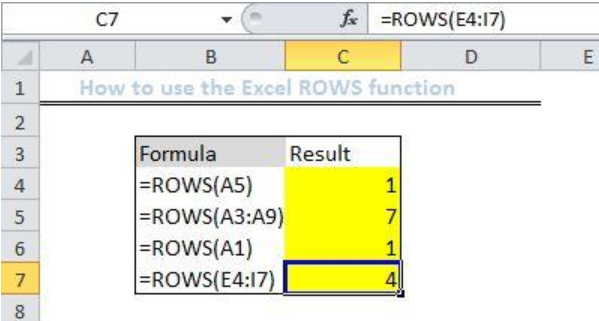Figure 1: Results of using the Excel ROWS function

## General Formula

`=ROWS(array)`

## Formula

`=ROWS(A5)`

## Setting up the Data

• We will set up a hypothetical list of arrays called Formula in column B
• Convert formula to text to see only the formula by right clicking on the cell and selecting “format text”.
• Column C will contain our results after we input the array formula extracted from each cell in Column B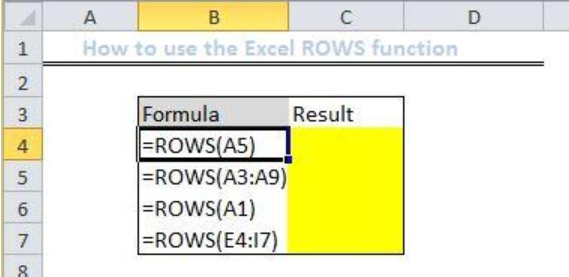Figure 2: Setting up the Data

## Using the Excel ROWS function

• We will click on Cell C4 and enter the array as displayed in Cell B4.
`=ROWS(A5)`
• We will press the enter key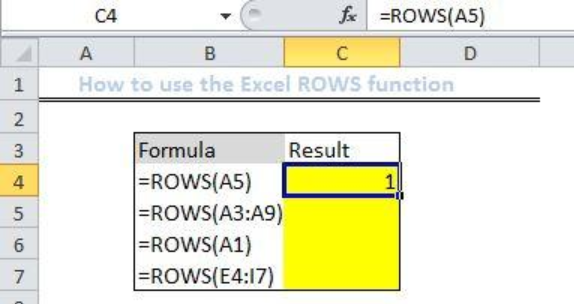Figure 3: Applying the ROWS function

• Next, we will select Cell C5
• We will enter the array displayed in Cell B5 into Cell C5
`=ROWS(A3:A9)`
• We will press the enter key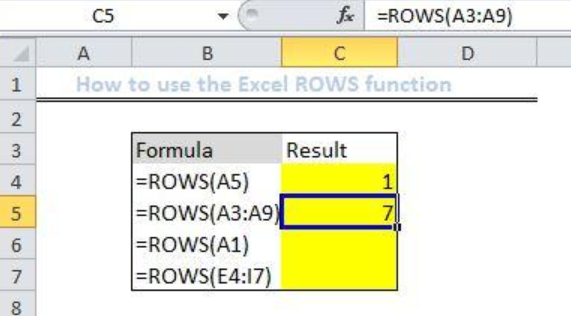Figure 4: Applying the ROWS function

• We will follow the steps above to find the results for the remaining cells.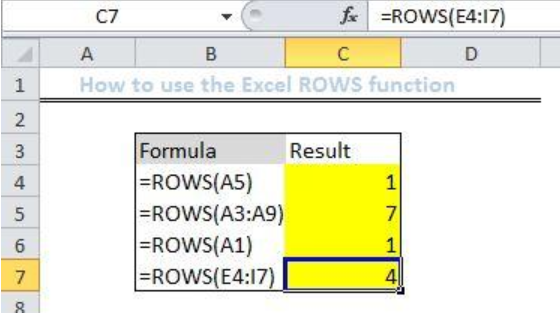Figure 5: Results of using the Excel ROWS function

## Explanation

The Excel ROWS Function will count the number of rows in the given reference. For instance, the Cell B5 contains ROWS(A3:A9), when we apply the ROWS function to this cell, it will return with seven because the range contains seven rows.

## Instant Connection to an Expert through our Excelchat Service

Most of the time, the problem you will need to solve will be more complex than a simple application of a formula or function. If you want to save hours of research and frustration, try our live Excelchat service! Our Excel Experts are available 24/7 to answer any Excel question you may have. We guarantee a connection within 30 seconds and a customized solution within 20 minutes.

### Did this post not answer your question? Get a solution from connecting with the expert.Another blog reader asked this question today on Excelchat:## Subscribe to Excelchat.coAnother blog reader asked this question today on Excelchat: